The goal of r2dii.plot is to help you plot 2DII data in an informative, beautiful, and easy way. It is designed to work smoothly with other “r2dii” packages – r2dii.data, r2dii.match, and r2dii.analysis. It also plays well with the ggplot2 package, which helps you customize your plots.

library(ggplot2)
library(dplyr, warn.conflicts = FALSE)
library(r2dii.data)
library(r2dii.match)
library(r2dii.analysis)
library(r2dii.plot)

Your data should have a structure similar to that of the demo datasets in the r2dii.data package.

loanbook <- loanbook_demo
ald <- ald_demo
#> Warning: The dataset ald_demois superseded as of r2dii.data 0.3.0 (expected July 2022).
#> Please use abcd_demo instead.
#> https://2degreesinvesting.github.io/posts/2022-03-02-ald-becomes-abcd/
scenario <- co2_intensity_scenario_demo
region <- region_isos_demo

Your workflow involves functions from the packages r2dii.match and r2dii.analysis.

matched <- loanbook %>%
match_name(ald) %>%
prioritize() # Remember to validate matches (see ?prioritize)

r2dii.plot supports three kinds of plots:

• Emission intensity,
• Techmix,
• Volume trajectory.

Each plot can be created using two types of functions:

• qplot_*() for standardized plots,
• plot_*() for ‘bare’ plots leaving the customization to the user.

For each kind, you’ll need to subset the specific rows you want to plot (for example with subset() or dplyr:filter()). For details see the documented “Requirements” of the argument data of each plot_*() or qplot_*() function (e.g. see ?plot_emission_intensity). If you forget to meet the data requirements the error message should guide you.

## ‘Quick’ plots

Quick plots allow you to create a good looking plot without extensive knowledge of other R packages. They are perfect to have a quick look into the data and for users who are interested in standard PACTA visualizations. Their common characteristics are:

• 5 year time span.
• Formatted data labels.
• Formatted axis labels and scales.
• A title (and a subtitle).

Use qplot_emission_intensity() with sda-like data.

data <- matched %>%
target_sda(ald, co2_intensity_scenario = scenario, region_isos = region) %>%
filter(
sector == "cement",
region == "global",
scenario_source == "demo_2020"
)
#> Warning: Removing abcd rows where emission_factor is NA

qplot_emission_intensity(data)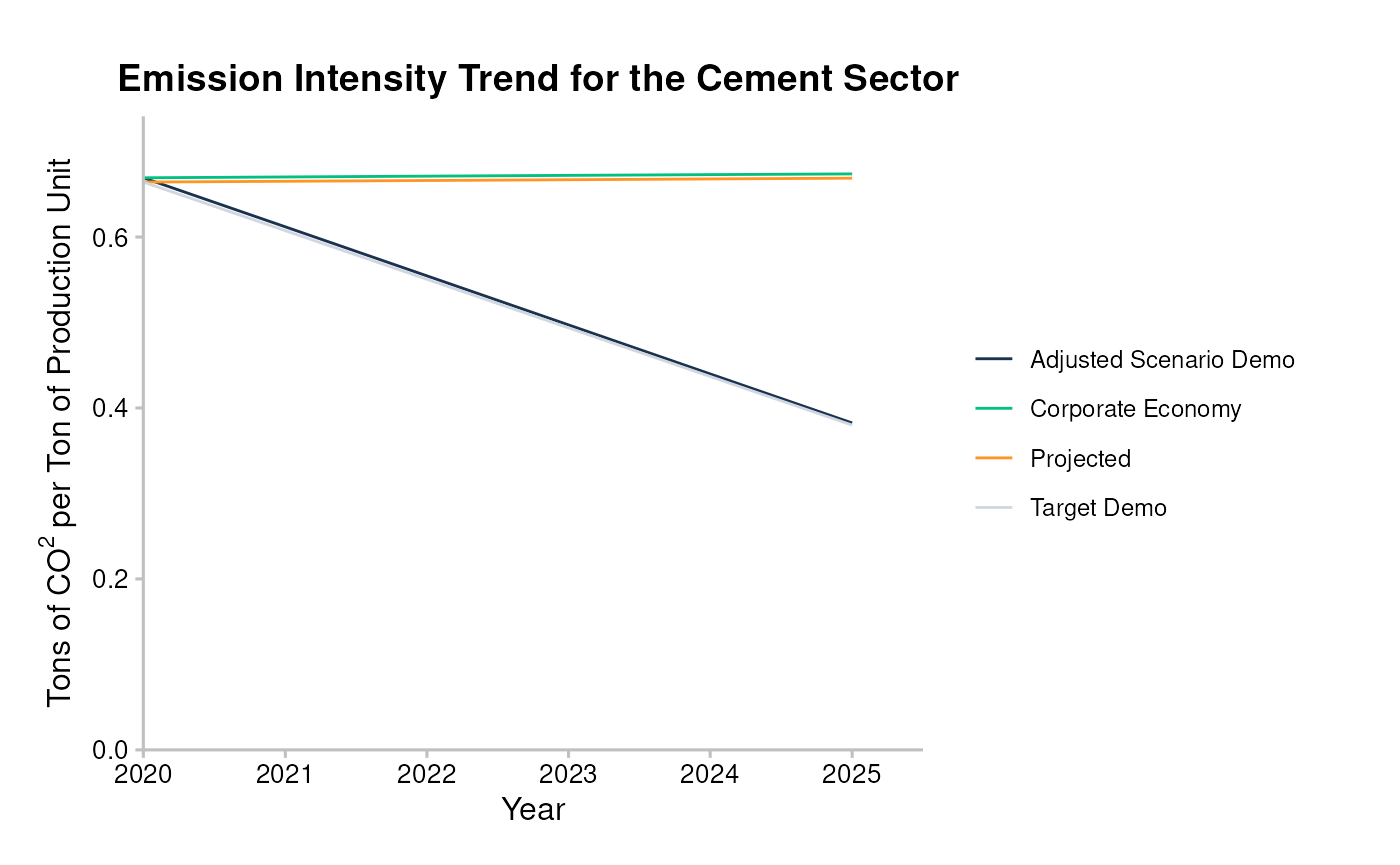Use qplot_trajectory() with market_share-like data.

data <- matched %>%
target_market_share(ald, scenario = scenario_demo_2020, region_isos = region) %>%
filter(
technology == "renewablescap",
region == "global",
scenario_source == "demo_2020"
)

qplot_trajectory(data)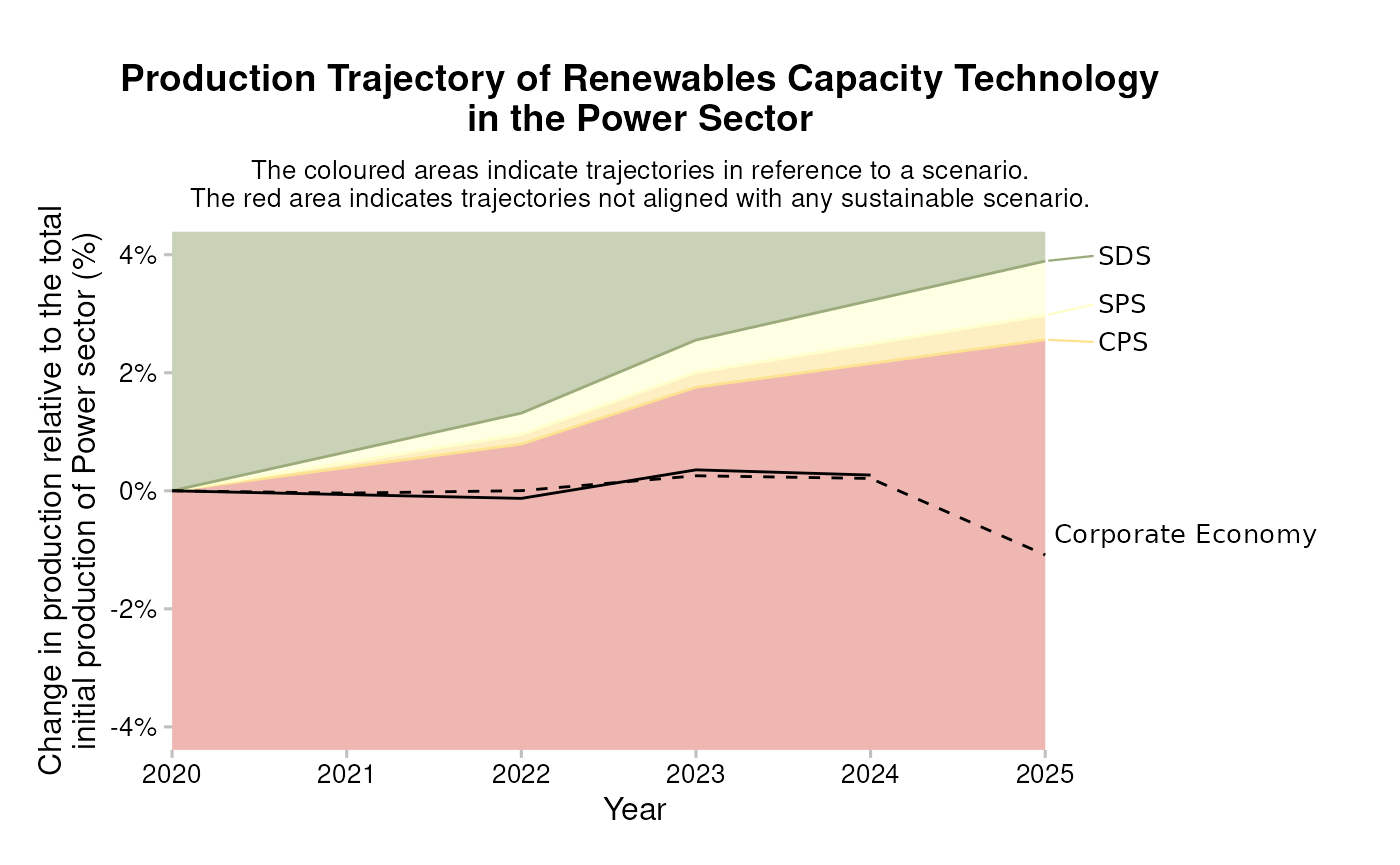Use qplot_techmix() with market_share-like data.

data <- matched %>%
target_market_share(ald, scenario = scenario_demo_2020, region_isos = region) %>%
filter(
sector == "power",
region == "global",
scenario_source == "demo_2020",
metric %in% c("projected", "corporate_economy", "target_sds")
)

qplot_techmix(data)
#> The technology_share values are plotted for extreme years.
#> Do you want to plot different years? E.g. filter data with:subset(data, year %in% c(2020, 2030)).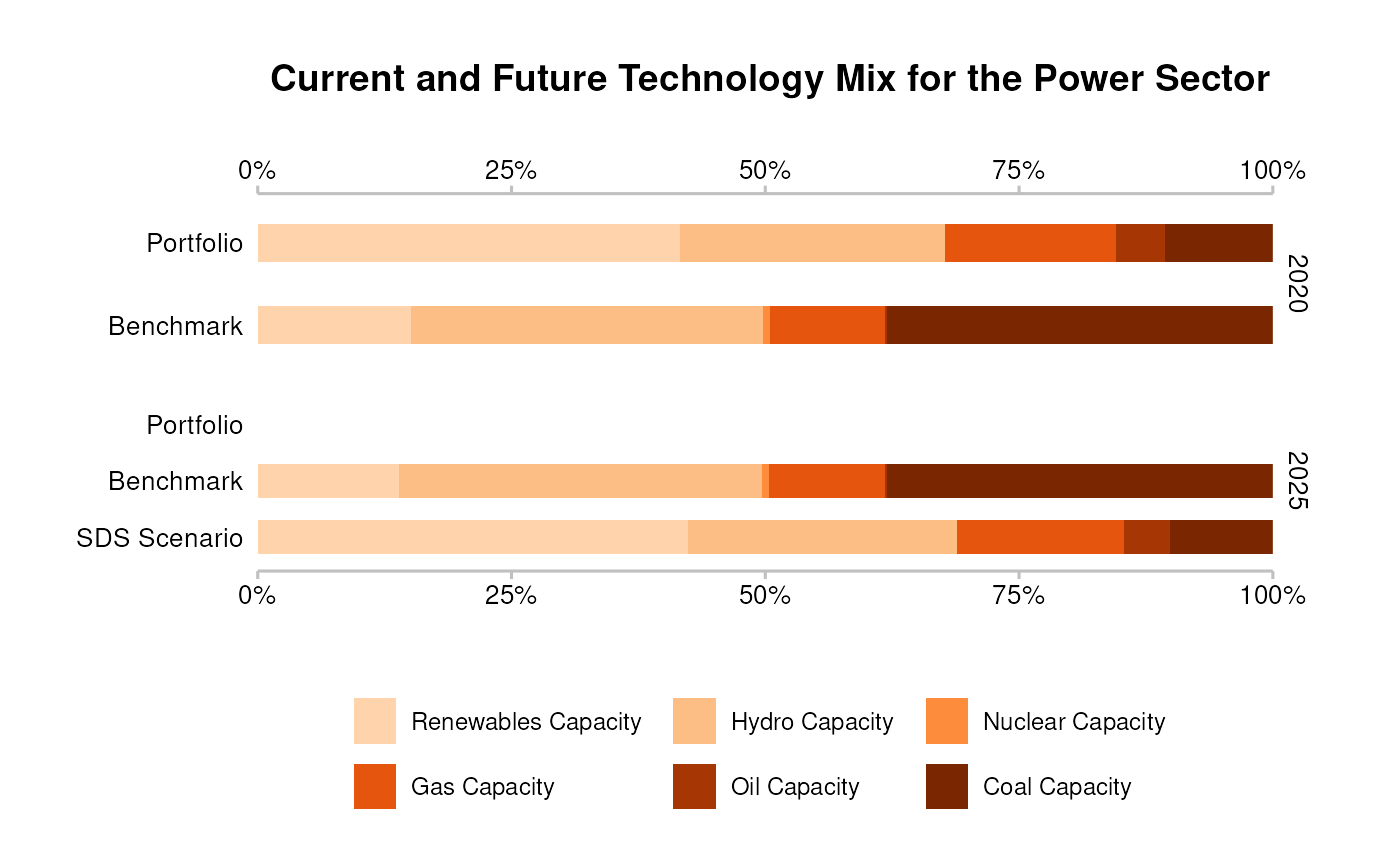## Plots

Plots created with plot_*() functions show the data as they are. To customize these plots you can use three strategies:

1. Use parameters of plot_*() functions,
2. Modify the input data,
3. Use ggplot2 functions.

The following sub sections show the three strategies applied to different functions of the plot_*() family.

### Plot customization strategies 1 and 3: parameters and ggplot2 functions

We will show how you might customize a plot using strategy 1 and 3 based on plot_emission_intensity().

The basic output of the function looks rather unappealing.

data <- matched %>%
target_sda(ald, co2_intensity_scenario = scenario, region_isos = region) %>%
filter(
sector == "cement",
region == "global",
scenario_source == "demo_2020"
)
#> Warning: Removing abcd rows where emission_factor is NA

plot_emission_intensity(data)
#> Warning: The data argument of plot_emission_intensity() must be prepped already as of r2dii.plot 0.4.0.
#> • From the next release you will need to call r2dii.plot::plot_emission_intensity(data)
#> prior to calling r2dii.plot::plot_emission_intensity().
#> • Alternatively custom data preparation will also become possible.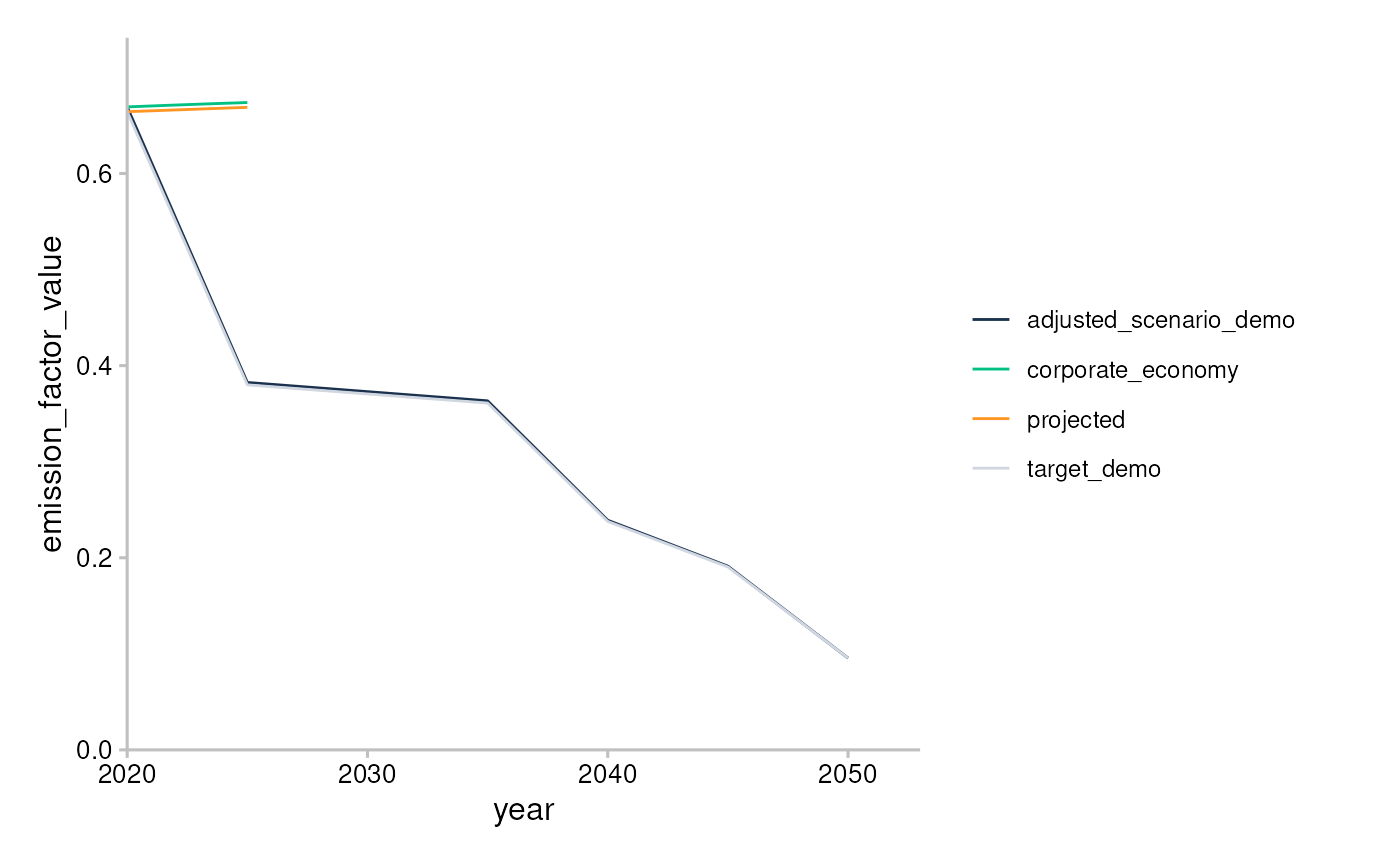You can use parameters of plot_emission_intensity() to replicate features of qplot_emission_intensity()(strategy 1), and add plot labels with ggplot2::labs() (strategy 3).

data <- matched %>%
target_sda(ald, co2_intensity_scenario = scenario, region_isos = region) %>%
filter(
sector == "cement",
region == "global",
scenario_source == "demo_2020"
)
#> Warning: Removing abcd rows where emission_factor is NA

plot_emission_intensity(data, convert_label = to_title, span_5yr = TRUE) +
labs(
title = "Emission Intensity Trend for the Cement Sector",
x = "Year",
y = "Tons of CO2 per Ton of Production Unit"
)
#> Warning: The data argument of plot_emission_intensity() must be prepped already as of r2dii.plot 0.4.0.
#> • From the next release you will need to call r2dii.plot::plot_emission_intensity(data)
#> prior to calling r2dii.plot::plot_emission_intensity().
#> • Alternatively custom data preparation will also become possible.### Plot customization strategies 2 and 3: input data and ggplot2 functions

You can also polish your plot by modifying the input data (strategy 2), for example:

• Changing the time span.
• Adding custom labels by adding a column ‘label’ or ‘label_tech’ to market_share-like data.

And by modifying output ggplot object (strategy 3), for example:

• Adding a title and a subtitle using ggplot2::labs().
• Changing x and y axis labels using ggplot2::labs().
• Customizing the colours and legend labels with ggplot2::scale_colour_manual() or r2dii.plot::scale_*() functions (see Styling functions).

Here is how you might customize each of the three kinds of plots using strategy 2 and 3:

• plot_emission_intensity() - add custom title and axis labels and modify colours and legend labels.
data <- sda %>%
filter(
sector == "cement",
region == "global",
scenario_source == "demo_2020",
year <= 2030
)

plot_emission_intensity(data) +
labs(
title = "Emission intensity plot for cement",
x = "Time",
y = "Tons of CO2 per ton of cement produced"
) +
scale_color_manual(
values = c("#4a5e54", "#a63d57", "#78c4d6", "#f2e06e"),
labels = c("Proj.", "Corp. Economy", "Target (demo)", "Adj. Scenario (demo)")
)
#> Warning: The data argument of plot_emission_intensity() must be prepped already as of r2dii.plot 0.4.0.
#> • From the next release you will need to call r2dii.plot::plot_emission_intensity(data)
#> prior to calling r2dii.plot::plot_emission_intensity().
#> • Alternatively custom data preparation will also become possible.
#> Scale for 'colour' is already present. Adding another scale for 'colour',
#> which will replace the existing scale.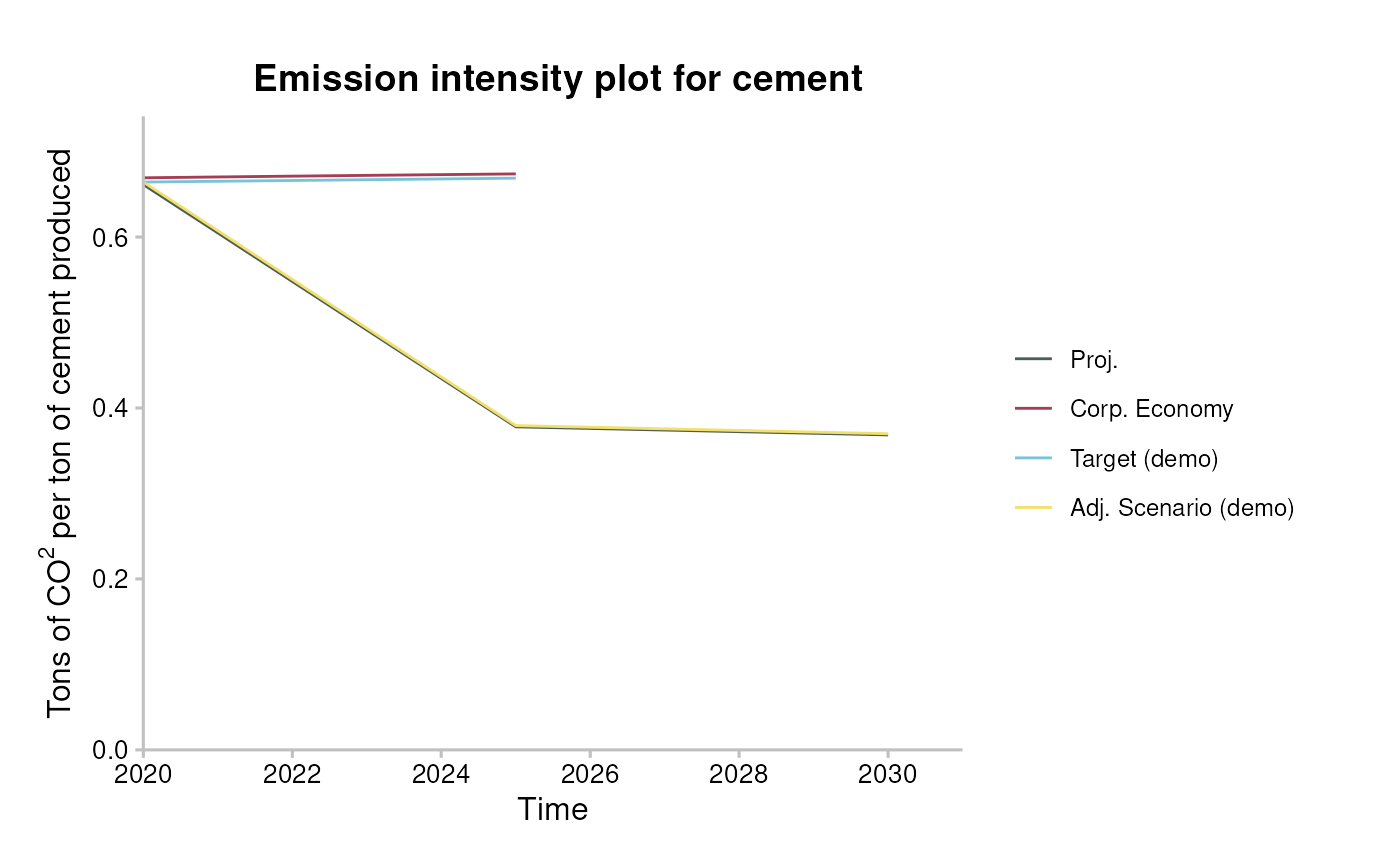• plot_trajectory() - change time span, add ‘label’ column and add custom title and axis labels.
data <- matched %>%
target_market_share(ald, scenario = scenario_demo_2020, region_isos = region) %>%
filter(
technology == "renewablescap",
region == "global",
scenario_source == "demo_2020",
year <= 2030
) %>%
mutate(
label = case_when(
metric == "projected" ~ "Your Portfolio",
metric == "corporate_economy" ~ "Benchmark (Corp. Economy)",
metric == "target_sds" ~ "Sustainable Development Scen.",
metric == "target_sps" ~ "Stated Policies Scen.",
metric == "target_cps" ~ "Current Policy Scen.",
TRUE ~ metric
)
)

plot_trajectory(data) +
scale_x_continuous(n.breaks = 3) +
labs(
title = "Portfolio Scenario Alignment for Renewables Technology",
x = "Year",
y = "Production normalized to start year"
) +
theme(plot.margin = unit(c(0.5, 6, 0.5, 1), "cm")) #so the long labels fit
#> Warning: The data argument of plot_trajectory() must be prepped already as of r2dii.plot 0.4.0.
#> • From the next release you will need to call r2dii.plot::plot_trajectory(data)
#> prior to calling r2dii.plot::plot_trajectory().
#> • Alternatively custom data preparation will also become possible.
#> Scale for 'x' is already present. Adding another scale for 'x', which will
#> replace the existing scale.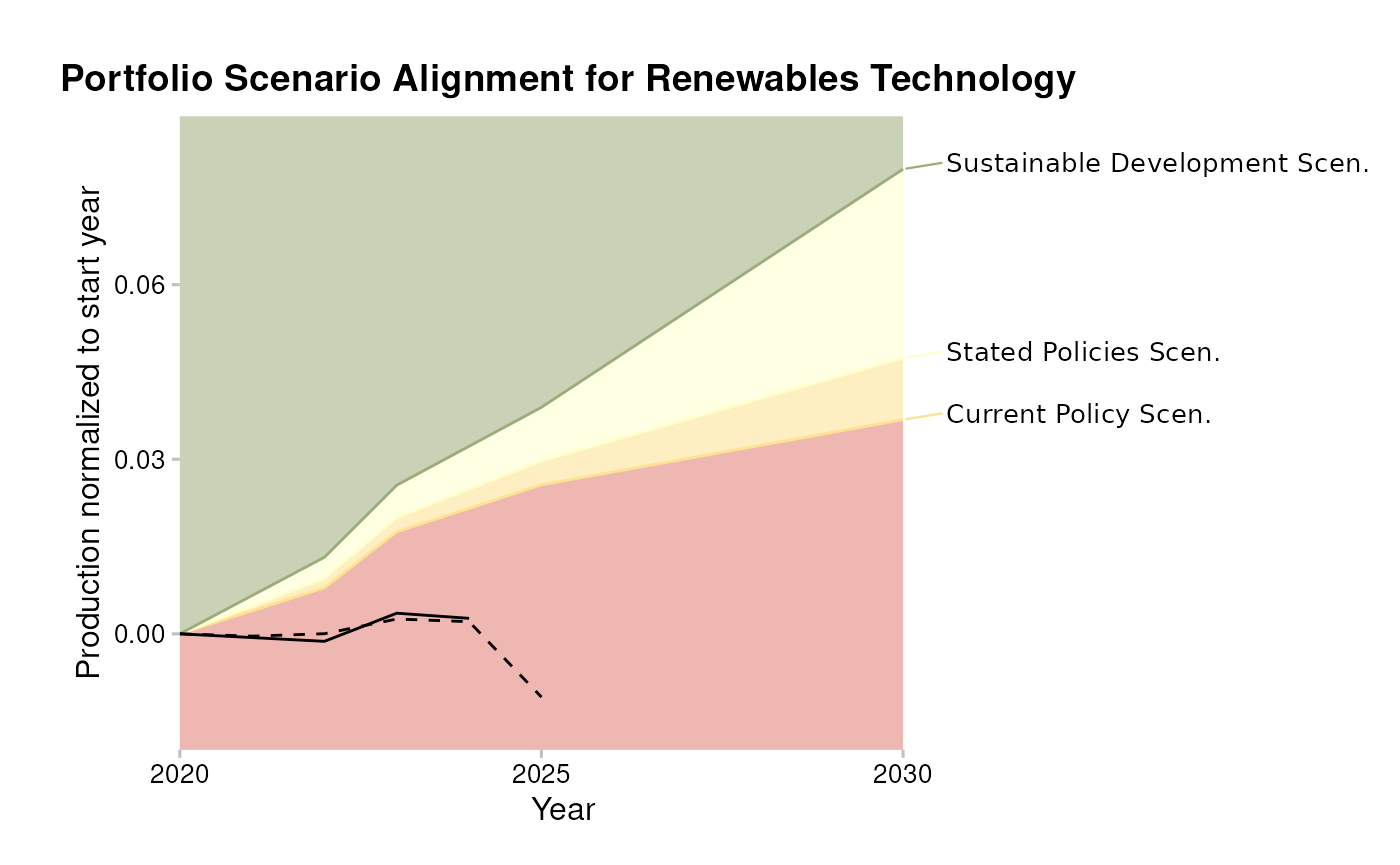• plot_techmix() - change time span, add ‘label’ column, apply custom colours and modify legend labels.
data <- market_share %>%
filter(
metric %in% c("projected", "corporate_economy", "target_sds"),
sector == "power",
scenario_source == "demo_2020",
region == "global",
year >= 2021,
year <= 2040 # custom time range
) %>%
mutate(
label = case_when(
metric == "projected" ~ "Your Portfolio",
metric == "corporate_economy" ~ "Corporate Economy Benchmark",
metric == "target_sds" ~ "SDS Scenario"
)
)

plot_techmix(data) +
scale_fill_manual(
values = c("black", "brown", "grey", "yellow", "blue", "green4"),
labels = paste(c("Coal", "Oil", "Gas", "Nuclear", "Hydro", "Renewables"), "Cap.")
)
#> Warning: The data argument of plot_techmix() must be prepped already as of r2dii.plot 0.4.0.
#> • From the next release you will need to call r2dii.plot::plot_techmix(data)
#> prior to calling r2dii.plot::plot_techmix().
#> • Alternatively custom data preparation will also become possible.
#> The technology_share values are plotted for extreme years.
#> Do you want to plot different years? E.g. filter data with:subset(data, year %in% c(2020, 2030)).
#> Scale for 'fill' is already present. Adding another scale for 'fill', which
#> will replace the existing scale.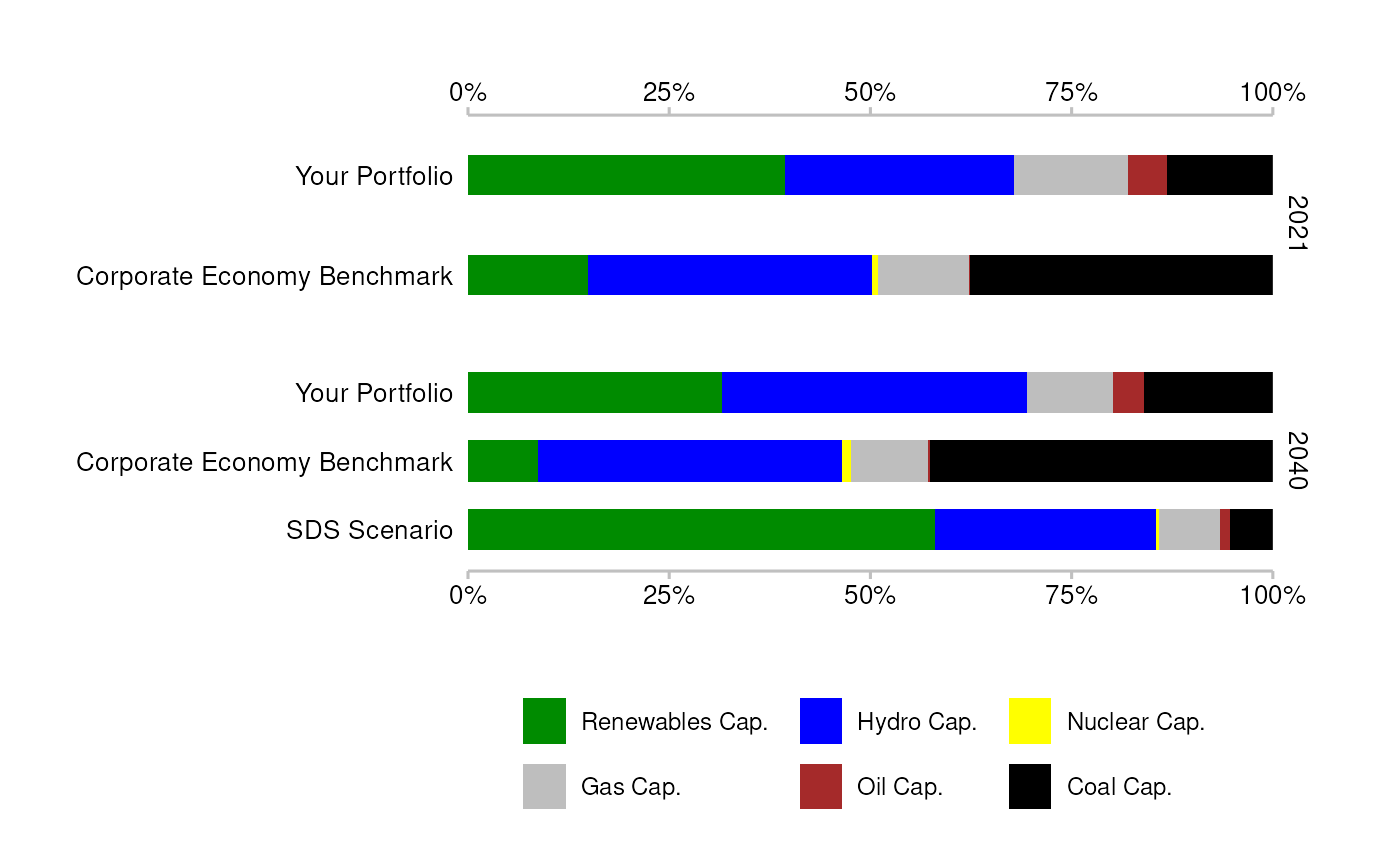• plot_techmix() - remove scenario data for start year.
data <- market_share %>%
filter(
metric %in% c("projected", "corporate_economy", "target_sds"),
sector == "power",
region == "global",
scenario_source == "demo_2020"
) %>%
filter(
!((metric == "target_sds") & (year == 2020))
)

plot_techmix(data)
#> Warning: The data argument of plot_techmix() must be prepped already as of r2dii.plot 0.4.0.
#> • From the next release you will need to call r2dii.plot::plot_techmix(data)
#> prior to calling r2dii.plot::plot_techmix().
#> • Alternatively custom data preparation will also become possible.
#> The technology_share values are plotted for extreme years.
#> Do you want to plot different years? E.g. filter data with:subset(data, year %in% c(2020, 2030)).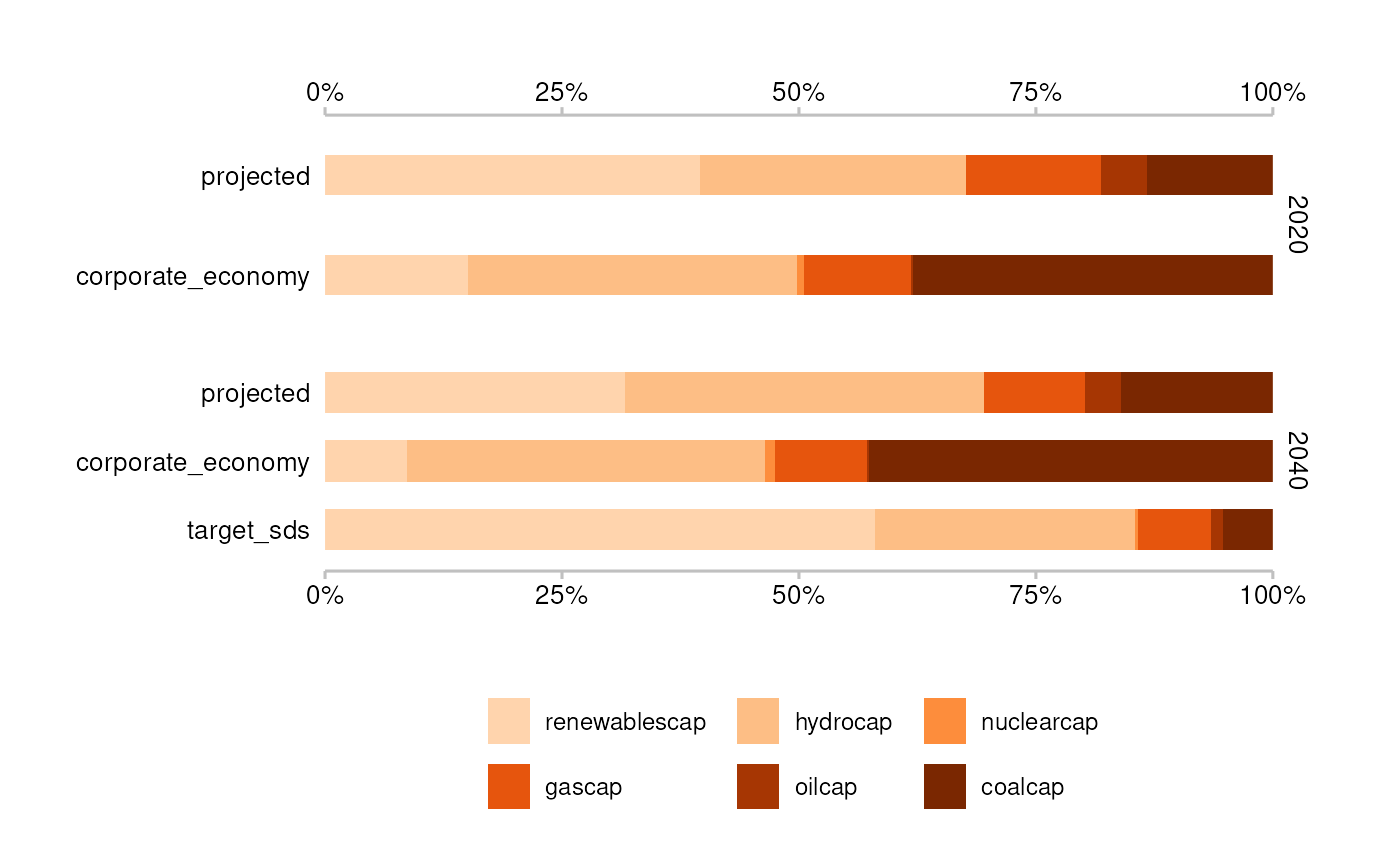## Styling functions

A number of functions allow you to customize any of your plots using the 2DII style:

• Use theme_2dii() to change the display of non-data content.
data <- market_share %>%
filter(
metric == "projected",
sector == "power",
region == "global",
year %in% c(2020, 2025)
)

ggplot(data, aes(x = factor(year), y = production)) +
geom_col() +
facet_wrap(~technology) +
theme_2dii()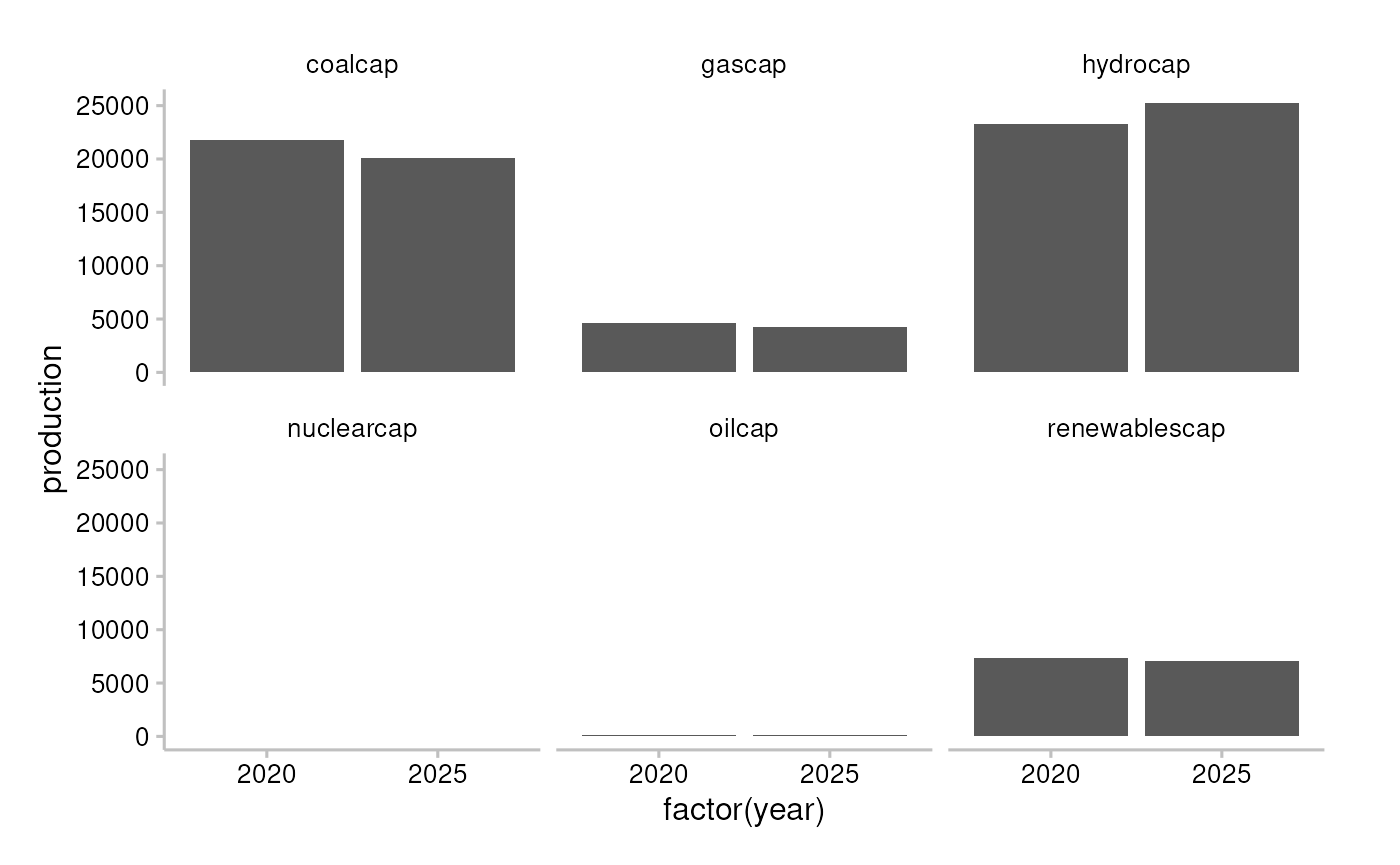• Use scale_colour_r2dii() and scale_fill_r2dii() to apply the 2DII colour palette (see ?scale_colour_2dii() to find out what are the available colour labels).
data <- market_share %>%
filter(
metric != "corporate_economy",
sector == "power",
region == "global",
technology == "renewablescap"
)

ggplot(data, aes(x = year, y = production, color = metric)) +
geom_line() +
scale_colour_r2dii(labels = c("dark_blue", "green", "orange", "ruby_red")) +
theme_2dii()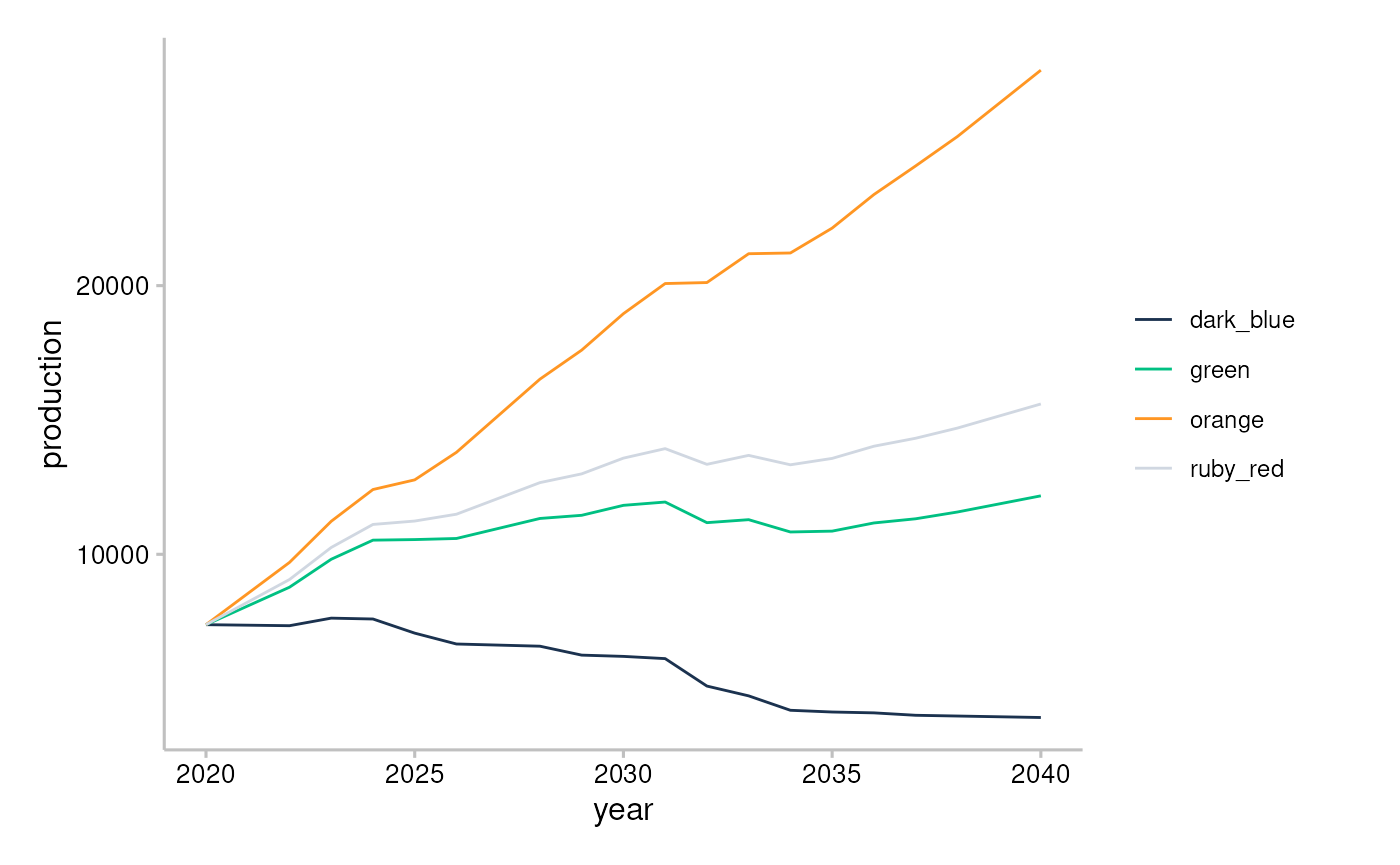• Use scale_colour_r2dii_sector() and scale_fill_r2dii_sector() to apply the 2DII colour palette for sectors (see ?scale_colour_2dii_sector() to find out what are the available colour labels).
data <- market_share %>%
filter(
metric == "projected",
region == "global",
year %in% c(2020, 2025)
) %>%
group_by(sector, year) %>%
summarise(production = sum(production))
#> summarise() has grouped output by 'sector'. You can override using the
#> .groups argument.

ggplot(data, aes(x = factor(year), y = production, fill = sector)) +
geom_col() +
scale_fill_r2dii_sector(sectors = c("automotive", "oil&gas", "power")) +
theme_2dii() +
facet_wrap(~sector)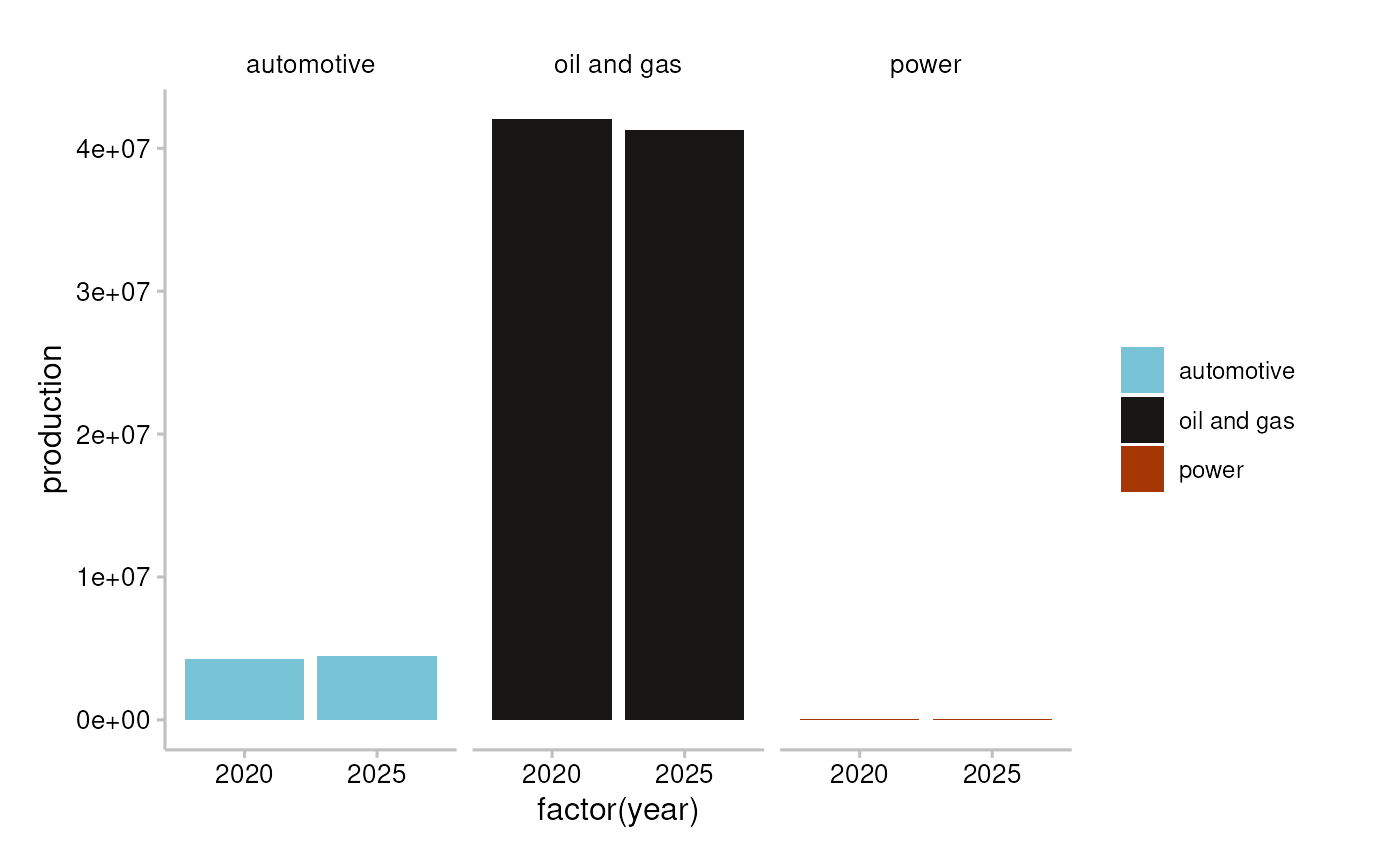• Use scale_colour_r2dii_tech() and scale_fill_r2dii_tech() to apply the 2DII colour palette for technologies (see ?scale_colour_2dii_tech() to find out what are the available colour labels).
technologies <- c("coalcap", "oilcap", "gascap", "hydrocap", "renewablescap")

data <- market_share %>%
filter(
metric == "projected",
sector == "power",
region == "global",
year %in% c(2020, 2025)
) %>%
mutate(technology = factor(technology, levels = technologies)) %>%
arrange(technology)

ggplot(data, aes(x = factor(year), y = production, fill = technology)) +
geom_col() +
scale_fill_r2dii_tech("power", technologies) +
facet_wrap(~technology) +
theme_2dii()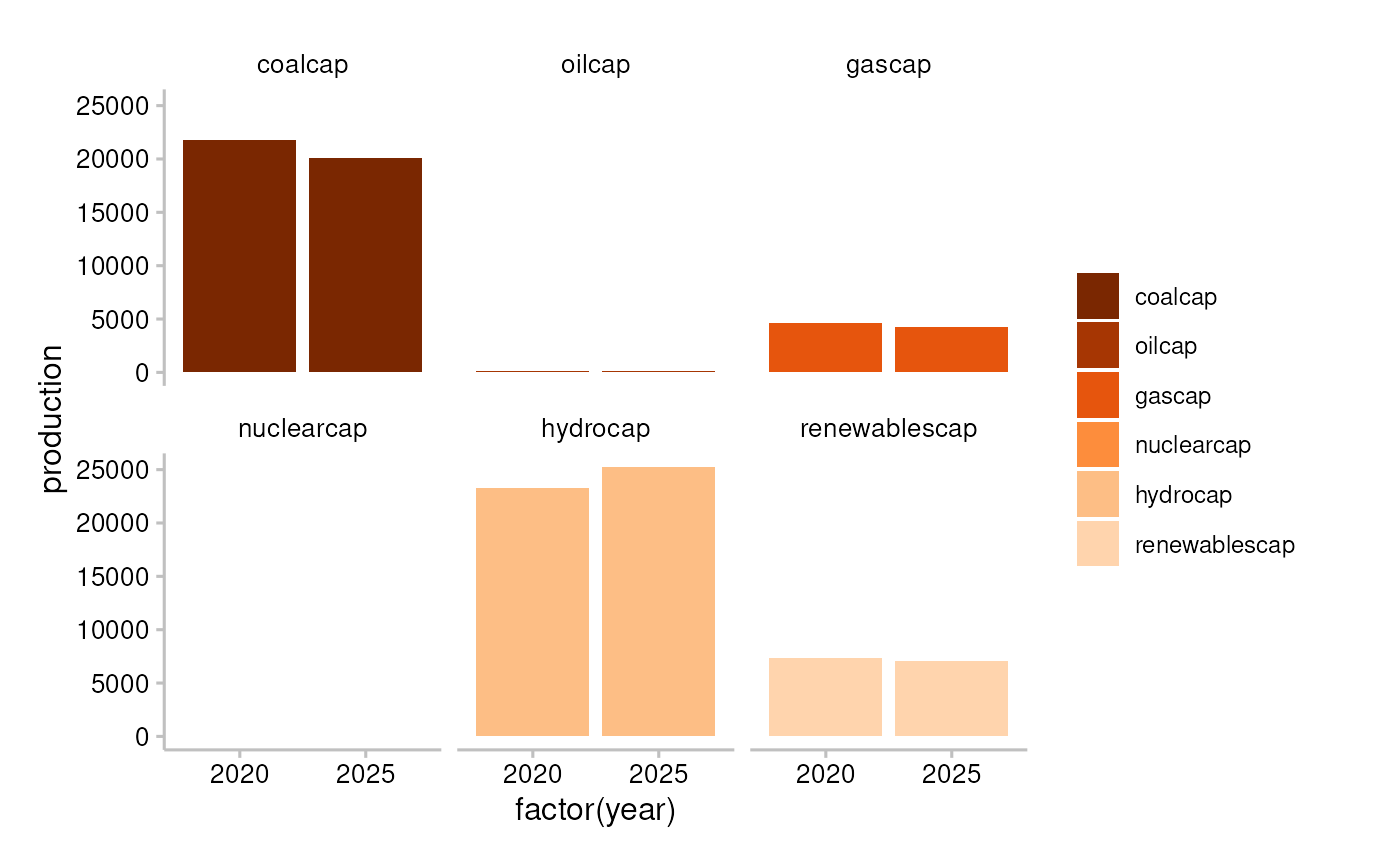## Funding

This project has received funding from the European Union LIFE program and the International Climate Initiative (IKI). The Federal Ministry for the Environment, Nature Conservation and Nuclear Safety (BMU) supports this initiative on the basis of a decision adopted by the German Bundestag. The views expressed are the sole responsibility of the authors and do not necessarily reflect the views of the funders. The funders are not responsible for any use that may be made of the information it contains.